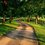# [Differential Geometry] How do we derive the Mercator's Projection of a sphere?

We begin with the familiar spherical parametrisation of the unit sphere $$X: U \subset \mathbb{R}^2 \rightarrow \mathbb{R}^3$$, where $X(\theta, \varphi) = (\sin{\theta}\cos{\varphi}, \sin{\theta}\sin{\varphi}, \cos{\theta}),$ is a diffeomorphic map from an open neighbourhood $U = \{(\theta, \varphi) \in \mathbb{R}^2; 0<\theta<\pi, 0<\varphi<2\pi \},$ which is obtained by rotating a semicircle on the xz-plane around the z-axis.

Interestingly, by making the change of coordinate, $u = \log \tan{\frac{1}{2}\theta}$ and $v=\varphi,$

we can show that a new parametrisation of the coordinate neighbourhood $X(U)$ can be derived.

First, we will start by putting $a= \tan{\frac{1}{2}\theta}$. Then, $X(\theta, \varphi) = (\sin{\theta}\cos{\varphi}, \sin{\theta}\sin{\varphi}, \cos{\theta}) = \left(\frac{2a}{1+a^2}\cos{\varphi}, \frac{2a}{1+a^2}\sin{\varphi}, \frac{1-a^2}{1+a^2} \right),$ where we have used the half-angle formulae for our tangent function.

Now $u= \tan{\frac{1}{2}\theta} \implies exp^u = \tan{\frac{1}{2}\theta} = a$ so

$=\left( \frac{2e^u}{1+e^{2u}} \cos{\varphi}, \frac{2e^u}{1+e^{2u}} \sin{\varphi}, \frac{1-e^{2u}}{1+e^{2u}}\right)$ $= \left( \frac{2}{e^{-u}+e^u} \cos{\varphi}, \frac{2}{e^{-u}+e^u} \sin{\varphi}, \frac{e^{-u}-e^u}{e^{-u}+e^u} \right)$ $= ( sech u, \cos v, sech u sin v, tanh u).$

Hence, $Y(u,v) = \left( sech (u), \cos(v), sech{u} \sin(v), \tanh(u)\right)$ gives a diffeomorphic map that parametrises the unit sphere.

In fact, we can also show that the coefficients of the first fundamental form in the parametrisation $Y$ are isothermal, hence locally conformal to an open neighbourhood of $\mathbb{R}^2$.

Now that you know that $y^{-1} : S^2 \rightarrow \mathbb{R}^3$ is a conformal (angle-preserving) mapping, what can you say about the meridians (lines of constant longitude) of the unit sphere, mapped under the Mercator's projection?Note by Bright Glow
3 years, 2 months ago

This discussion board is a place to discuss our Daily Challenges and the math and science related to those challenges. Explanations are more than just a solution — they should explain the steps and thinking strategies that you used to obtain the solution. Comments should further the discussion of math and science.

When posting on Brilliant:

• Use the emojis to react to an explanation, whether you're congratulating a job well done , or just really confused .
• Ask specific questions about the challenge or the steps in somebody's explanation. Well-posed questions can add a lot to the discussion, but posting "I don't understand!" doesn't help anyone.
• Try to contribute something new to the discussion, whether it is an extension, generalization or other idea related to the challenge.

MarkdownAppears as
*italics* or _italics_ italics
**bold** or __bold__ bold
- bulleted- list
• bulleted
• list
1. numbered2. list
1. numbered
2. list
Note: you must add a full line of space before and after lists for them to show up correctly
paragraph 1paragraph 2

paragraph 1

paragraph 2

[example link](https://brilliant.org)example link
> This is a quote
This is a quote
    # I indented these lines
# 4 spaces, and now they show
# up as a code block.

print "hello world"
# I indented these lines
# 4 spaces, and now they show
# up as a code block.

print "hello world"
MathAppears as
Remember to wrap math in $$ ... $$ or $ ... $ to ensure proper formatting.
2 \times 3 $2 \times 3$
2^{34} $2^{34}$
a_{i-1} $a_{i-1}$
\frac{2}{3} $\frac{2}{3}$
\sqrt{2} $\sqrt{2}$
\sum_{i=1}^3 $\sum_{i=1}^3$
\sin \theta $\sin \theta$
\boxed{123} $\boxed{123}$

Sort by:

Given a sphere with a map on it, put it inside of a cylinder of the same diameter (usually aligned with the axis of the Earth), draw a ray from the center of the sphere through a given point on the map, and continue until it intersects the cylinder. Then a point on the map on the sphere will have been projected into a corresponding point on the cylinder. When all done, unroll the cylinder flat.

This is the reason why you never see the north or south pole of the Earth on a typical Mercator projection.

- 3 years, 2 months ago

Thanks for your comment, it's a very clever way of preserving angles on a planar map of the sphere!

- 3 years, 2 months ago

I realize now that you were just starting on this note when I answered. Obviously you know quite a bit about this subject. And I had forgotten about the fact Mercator projections preserves local angles, i.e., only the scaling changes locally at different latitudes, i.e. maps of cities look approximately normal except for size. That is an interesting property.

- 3 years, 2 months ago

Yes, I realised that too... It is indeed!

- 3 years, 2 months ago